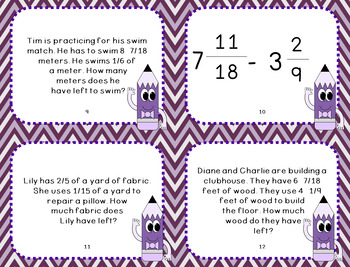# Subtracting Fractions with Unlike Denominators Task CardsSubject
Resource Type
File Type

PDF

(2 MB|25 pages)
Standards
• Product Description
• StandardsNEW

Looking for a new way to get students to review subtracting fractions with unlike denominators? These versatile task cards are a great addition to the classroom. Task cards can be used for review games like Scoot, math centers, independent practice, and more.

This packet contains:

+28 subtracting fractions with unlike denominators task cards

+minimal color edition of the task cards

+black and white printer-friendly task card set

+response sheet

Adding and Subtracting Fractions with Unlike Denominators Edition #1

St. Patrick's Day Add and Subtract Fractions with Unlike Denominators Puzzles

Summer Adding and Subtracting Fractions With Unlike Denominators Puzzles

Solve word problems involving addition and subtraction of fractions referring to the same whole, including cases of unlike denominators, e.g., by using visual fraction models or equations to represent the problem. Use benchmark fractions and number sense of fractions to estimate mentally and assess the reasonableness of answers. For example, recognize an incorrect result 2/5 + 1/2 = 3/7, by observing that 3/7 < 1/2.
Add and subtract fractions with unlike denominators (including mixed numbers) by replacing given fractions with equivalent fractions in such a way as to produce an equivalent sum or difference of fractions with like denominators. For example, 2/3 + 5/4 = 8/12 + 15/12 = 23/12. (In general, 𝘢/𝘣 + 𝘤/𝘥 = (𝘢𝘥 + 𝘣𝘤)/𝘣𝘥.)
Total Pages
25 pages
Included
Teaching Duration
N/A
Report this Resource to TpT
Reported resources will be reviewed by our team. Report this resource to let us know if this resource violates TpT’s content guidelines.